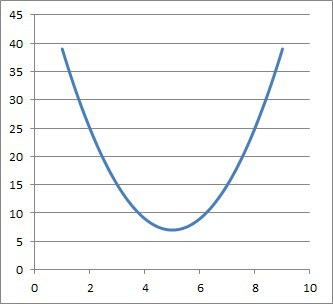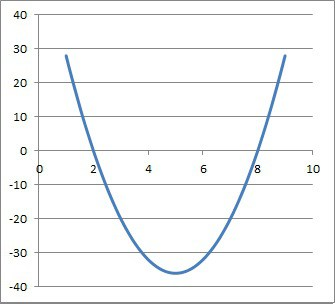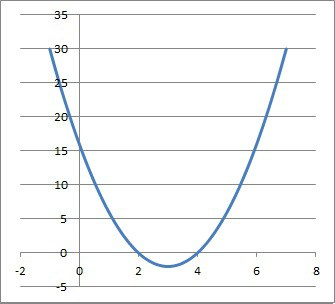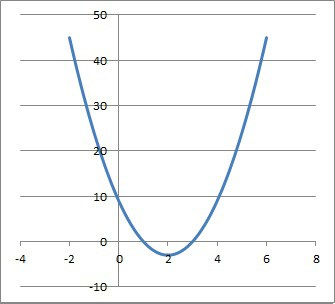# How To Find The Vertex Of A Parabola (3 Methods To Know)

The vertex of a parabola can tell us the extreme value (local maximum or minimum) of a parabola, along with the line of symmetry of the curve.  However, we still need to know how to find the vertex in a variety of situations.

So, how do you find the vertex of a parabola?  To find the vertex of a parabola, you can use the graph (find the maximum/minimum of the curve), use two points (using a parabola’s symmetry), or use the corresponding quadratic equation. You can find the vertex of a parabola from a quadratic equation in vertex form, factored form, or standard form.

Of course, given any three points on a parabola, we can always find the equation of the corresponding quadratic and use it to find the vertex.

In this article, we’ll talk about how to find the vertex of a parabola.  We’ll also show some examples to make the concepts clear.

Let’s get started.

Having math trouble?

Looking for a tutor?

## How To Find The Vertex Of A Parabola

There are a few ways you can find the vertex of a parabola:

• From an equation: if you have a quadratic equation in vertex form, factored form, or standard form, you can use it to find the vertex of the corresponding parabola.
• From two points (symmetry): if you have two points on a horizontal line that are an equal distance from the vertex of a parabola, you can use symmetry to find the vertex.
• From a graph: if you have the graph of a quadratic, you can find the local maximum (top of the curve) or local minimum (bottom of the curve) to find the vertex of the parabola.

Let’s start off with the first method: finding the vertex from an equation.

### How To Find The Vertex Of A Parabola From An Equation

The easiest way to find the vertex of a parabola from an equation is to convert it to vertex form or factored form.  However, we can convert any form of a quadratic equation into any other form.

The procedure is slightly different in each case, so let’s go through each one in turn.

#### How To Find The Vertex Of A Parabola In Vertex Form

To find the vertex of a parabola in vertex form, look at the constants h and k in the corresponding quadratic equation:

• y = a(x – h)2 + k

This form is easiest to find the vertex from, since all we need to do is read the coordinates from the equation.

The vertex will be the point (h, k).

#### Example: How To Find The Vertex Of A Parabola From An Equation In Vertex Form

Let’s say we have the following quadratic equation in vertex form:

• y = 2(x – 5)2 + 7

In this case, h = 5 and k = 7.  This means that the vertex of the corresponding parabola is (h, k) = (5, 7).

We can verify this with the graph below.The parabola y = 2(x – 5)2 + 7 has its vertex at the point (5, 7).

#### How To Find The Vertex Of A Parabola In Factored Form

Find the vertex of a parabola in factored form is a little more involved, but it is still not too difficult.  The steps are as follows:

• 1.) Find the two zeros (roots), r and s, of the quadratic from the factored form.  These value comes from the factored form y = a(x – r)(x – s).
• 2.) Take the average of r and s to get h = (r + s) / 2 (h is the x-coordinate of the vertex).
• 3.) Substitute x = h into the quadratic factored form to find y.  This will always give us a y-coordinate of k = -a(r – s)2 / 4
• 4.) The vertex is the point (h, k) = ((r + s) / 2, -a(r – s)2 / 4)

Let’s look at an example.

#### Example: How To Find The Vertex Of A Parabola From An Equation In Factored Form

Let’s say we have the following quadratic equation in factored form:

• y = 4(x – 2)(x – 8)

Step 1: In this case, r = 2 and s = 8 are the two zeros (roots) of this quadratic equation.

Step 2: The average of r = 2 and s = 8 is (2 + 8) / 2 = 5.  So, h = 5 is the x-coordinate of the vertex.

Step 3: We substitute x = 5 into the quadratic equation to get 4(5 – 2)(5 – 8) = 4(3)(-3) = -36.  So, k = -36 is the y-coordinate of the vertex.

Step 4: The vertex of the parabola is the point (h, k) = (5, -36).

We can verify this with the graph below.The parabola y = 24(x – 2)(x – 8) has its vertex at the point (5, -36).

Note: we can also convert the quadratic equation to vertex form and then read the coordinates as in the last example:

• y = 4(x – 2)(x – 8)
• y = 4(x2 – 8x – 2x + 16)  [used FOIL]
• y = 4(x2 – 10x + 16)  [combine like terms]
• y = 4(x2 – 10x + 25 – 25 + 16)  [complete the square for x2 + 10x by adding 25]
• y = 4((x – 5)2 – 9)  [factor x2 – 10x + 25 as a perfect square trinomial (x – 5)2]
• y = 4(x – 5)2 – 36

We get the same answer for the vertex: (5, -36).

Having math trouble?

Looking for a tutor?

#### How To Find The Vertex Of A Parabola In Standard Form

To find the vertex of a parabola in standard form, we can convert to either vertex form or factored form and then follow the steps in the previous examples.

We also have the option of using the shortcut formula for the vertex of a parabola in standard form.  If the quadratic has the equation

• y = ax2 + bx + c

then the vertex has an x-coordinate of –b/2a.  We can then substitute x = -b/2a into the quadratic equation to find the value of y.

This gives us a y-coordinate of c – (b2 / 4a) for the vertex of the parabola.

So, the coordinates of the vertex are (-b / 2a, c – (b2 / 4a)).

#### Example: How To Find The Vertex Of A Parabola From An Equation In Standard Form

Let’s say we have the following quadratic equation in standard form:

• y = 2x2 – 12x + 16

Let’s convert it to factored form and find the vertex that way:

• y = 2(x2 – 6x + 8)
• y = 2(x – 2)(x – 4)

So, r = 2 and s = 4.  Taking the average gives us (2 + 4) / 2 = 3.

The x-coordinate of the vertex is 3.  If we substitute this into the quadratic, we can find y:

• y = 2(x – 2)(x – 4)
• y = 2(3 – 2)(3 – 4)
• y = 2(1)(-1)
• y = -2

The y-coordinate of the vertex is -2.  So, the vertex of the parabola is (3, -2).

We can also find this with our formulas that involve a, b, and c (the coefficients of the quadratic equation in standard form).

First, the x-coordinate of the vertex:

• x = -b / 2a
• x = -(-12) / 2(2)
• x = 12 / 4
• x = 3

Now, the y-coordinate of the vertex:

• y = c – (b2 / 4a)
• y = 16 – ((-12)2 / 4(2))
• y = 16 – (144 / 8)
• y = 16 – 18
• y = -2

So, the vertex of the parabola is (3, -2), just as we found before. You can also see this in the graph below.The parabola y = 2x2 -12x + 16 has its vertex at the point (3, -2).

### How To Find The Vertex Of A Parabola From Two Points (Symmetry)

If we have two points on a horizontal line that are equidistant from the vertex, then we can find the x-coordinate.

This is due to the symmetry of a parabola about the line x = h (where h is the x-coordinate of the vertex).

Since a parabola has symmetry, a horizontal line y = d will intersect the parabola at two points that are an equal distance from the vertex (or from the line of symmetry).

We can use this to our advantage to take the average of these two x-coordinates to find the x-coordinate of the vertex.

#### Example: How To Find The Vertex Of A Parabola From Two Points (Symmetry)

Let’s say we have two points (3, 7) and (9, 7) that lie on a parabola.  Since the y-coordinates are the same (both are y = 7), we know that these two points lie on the same horizontal line (y = 7).

This means that the parabola will intersect this horizontal line at two points that are the same distance from the line of symmetry (the horizontal line x = h, which goes through the vertex).

Taking the average of the two x-values, we get (3 + 9) / 2 = 6.  So, the x-coordinate of the vertex is 6.

We would need more information to find the y-coordinate of the vertex (without more information, we don’t even know if the parabola is concave or convex!)

### How To Find The Vertex Of A Parabola From A Graph

To find the vertex of a parabola from a graph, all we need to do is take a look at where the curve has a local minimum (for a convex/concave up curve) or local maximum (for a concave/concave down curve).

This point (the local minimum or maximum) is the vertex of the parabola.

#### Example: How To Find The Vertex Of A Parabola From A Graph

Given the graph of the parabola y = 3x2 – 12x + 9 (pictured below):The parabola y = 3x2 -12x + 9 has its vertex at the point (2, -3).

We can see by visual inspection that the vertex is at (2, -3), since that point is the local minimum of the curve.

## Conclusion

Now you know  how to find the vertex of a parabola from an equation, from two points on a horizontal line, or from a graph.

You can learn about the focus of a parabola and what it means here.

You can learn how to find the domain and range of a parabola here.

You might also want to read my article on common questions about ellipses (a parabola is a conic section, just like an ellipse).